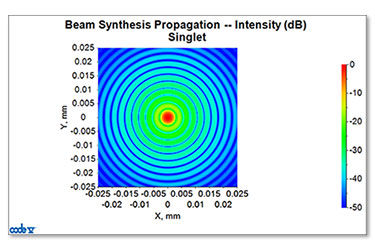## Quick Tip: Conditions and Assumptions in CODE V Diffraction Calculations

CODE V features many different methods of considering diffraction in optical systems. The PSF option for analyzing a point spread function, MTF for analyzing a modulation transfer function, and BSP for simulating general beam propagation are relied upon by many engineers working with systems where diffraction matters.All diffraction calculations in CODE V are based on equations that assume Kirchhoff boundary conditions (which, in turn, assume that the edge of an aperture is a perfect conductor, so that the E field goes to zero at the edge).1 Even though Kirchhoff boundary conditions are seldom met at optical frequencies, this is a reasonable assumption as long as the apertures are larger than about 10λ. For smaller apertures, edge effects become significant.

For features that are much larger than 10λ (>~100λ), then CODE V geometrical ray tracing tools are appropriate as waves can be approximated by straight lines. When features are smaller than 10λ, more computationally intensive methods that solve Maxwell’s equations are needed.2

Footnotes

1. Born & Wolf, Principles of Optics (see section 8.3.2 of the 4th edition ).
2. Synopsys RSoft products are applicable in these small wavelength regions.